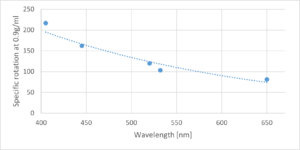## Physics experiments in undergraduate labs

18 May 2022

#### HEXA-BEAM Laser

If you are running physics experiments in your undergraduate labs that require to change different wavelengths, wouldn’t it be great to switch between the different wavelengths without the need to realign the laser set up from scratch?

The HEXA-BEAM Laser can do just that. This is done by rotating one single knob.

With one simple laser source, you can select your laser colour at the turn of a knob!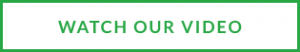## Physics Experiments with the HEXA-BEAM Laser

18 May 2022

Physics experiment for rotating the plane of polarisation (also known as Biot’s sugar experiment)

Biot’s optical rotation experiment demonstrates the effect of chiral media (sugar solution) on the plane of polarisation of linearly polarised light. This experiment allows for investigation into the effects of polarisation, chirality, scattering and fluorescence.

Chirality is the name given to the property of molecules and systems whose image cannot be superimposed upon its mirror image. When linearly polarised light is passed through a chiral medium, such as a sugar solution, the plane of polarisation of the incident light is rotated due to its asymmetric structure, creating bright and dark bands that can be observed along the optical path.

Linearly polarised light is composed of left and right circularly polarised partial light waves, with both components having equal amplitudes. When these partial waves propagate through the chiral material, they encounter different refractive indices due to the asymmetry of the medium. The partial wave that oscillates through a larger refractive index will travel slower than its counterpart, producing a phase difference between them. This phase difference causes the plane of polarisation to rotate. The angle of rotation caused by the differing refractive indices is described by the following equation: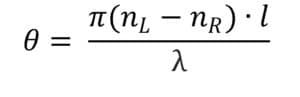Here,is the optical angle of rotation,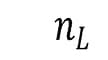andare the refractive indices of the left and right circularly polarised partial waves respectively,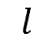is the length of the optical path andis the wavelength of the light beam.

The rotation of linearly polarised light causes observable bright and dark bands to appear along the cell containing the solution because the light is not entirely observable from one angle.

Equipment required

• HEXA-BEAM Laser

The HEXA-BEAM laser makes it easy to run this experiment at different colours, allowing for the student to appreciate the effect of wavelength on the experiment. With a knob at the back of the laser, students can quickly change wavelength without any realignment needed.

The HEXA-BEAM laser is aligned down the centre of the glass tube to reduce any scattering. The bands of minima/maxima are visible and their separation can be measured.

• Glass tube
• Rotatable polarising filter
• Observation sccreen
• Photon detector
• RuleAnalysis

The specific rotation can be determined by the distance between successive minima or maxima, as the distance between these points is equivalent to a specific rotation of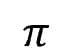.  Therefore, the specific rotation of the polarisation plane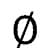can be calculated by adjusting the values in the below equationwhere L is the distance between the consecutive extrema that are measured, and C is the concentration of the solution the laser is passing through. The values of the specific rotation can then be compared to a mathematical fit using Drude’s expression.

Sources of error

• Inhomogeneity of solution – the presence of bubbles within the sugar solution will prevent accurate results being obtained
• Location of the maxima/minima
• Parallax error observing the maxima/minima due to the laser beam being contained within the glass tube
• Position of detector outside glass tube
• Scattering of laser beam entering the tube